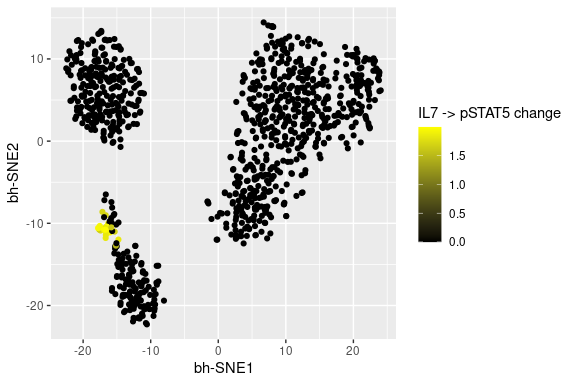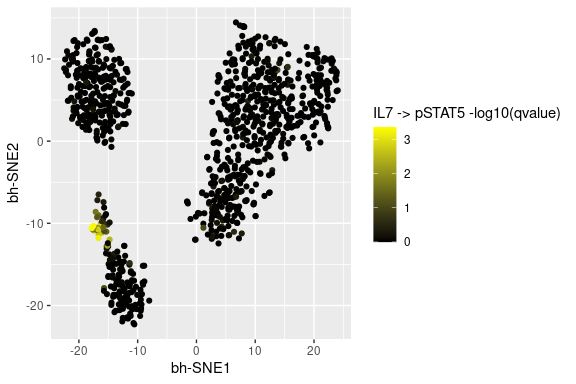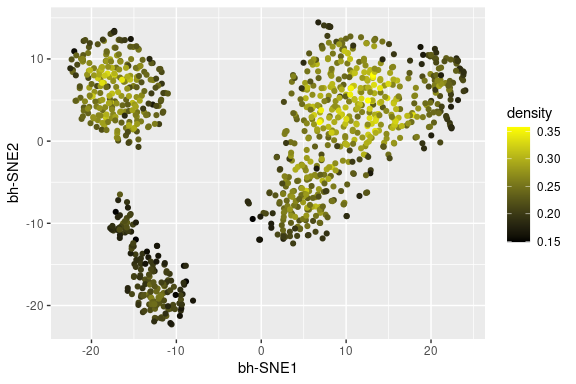# Step 3: Post-Processing

### The post-processing function:

This vignette covers what takes place following the generation of SCONE output detailed in TheSconeWorkflow.Rmd. The obvious step that needs to take place is the Scone generated columns being merged into the original input data. The user gets the option of log base 10 transforming q values, which is easier to visualize. The user also gets the option to run t-SNE on the data, such that said maps can be colored by SCONE generated values. In this case, t-SNE is run utilizing the Rtsne package, using the same markers that were used as input for the KNN. generation.

library(Sconify)
wand.final <- PostProcessing(scone.output = wand.scone,
cell.data = wand.combined,
input = input.markers)
## Read the 1000 x 27 data matrix successfully!
## OpenMP is working. 1 threads.
## Using no_dims = 2, perplexity = 30.000000, and theta = 0.500000
## Computing input similarities...
## Building tree...
## Done in 0.18 seconds (sparsity = 0.126334)!
## Learning embedding...
## Iteration 50: error is 63.709817 (50 iterations in 0.42 seconds)
## Iteration 100: error is 60.605314 (50 iterations in 0.23 seconds)
## Iteration 150: error is 60.180143 (50 iterations in 0.20 seconds)
## Iteration 200: error is 60.031427 (50 iterations in 0.20 seconds)
## Iteration 250: error is 59.955049 (50 iterations in 0.21 seconds)
## Iteration 300: error is 1.372635 (50 iterations in 0.15 seconds)
## Iteration 350: error is 1.257765 (50 iterations in 0.13 seconds)
## Iteration 400: error is 1.226973 (50 iterations in 0.13 seconds)
## Iteration 450: error is 1.216037 (50 iterations in 0.12 seconds)
## Iteration 500: error is 1.210123 (50 iterations in 0.13 seconds)
## Iteration 550: error is 1.204418 (50 iterations in 0.13 seconds)
## Iteration 600: error is 1.197492 (50 iterations in 0.12 seconds)
## Iteration 650: error is 1.194357 (50 iterations in 0.17 seconds)
## Iteration 700: error is 1.190561 (50 iterations in 0.16 seconds)
## Iteration 750: error is 1.186697 (50 iterations in 0.14 seconds)
## Iteration 800: error is 1.184417 (50 iterations in 0.17 seconds)
## Iteration 850: error is 1.182298 (50 iterations in 0.17 seconds)
## Iteration 900: error is 1.181342 (50 iterations in 0.18 seconds)
## Iteration 950: error is 1.179848 (50 iterations in 0.16 seconds)
## Iteration 1000: error is 1.178311 (50 iterations in 0.12 seconds)
## Fitting performed in 3.42 seconds.
wand.combined # input data
## # A tibble: 1,000 × 51
##    CD3(Cd110)Di CD3(Cd111)Di CD3(Cd112)Di CD235-61-7-15(… CD3(Cd114)Di
##             <dbl>          <dbl>          <dbl>             <dbl>          <dbl>
##  1       -0.245          -0.291          -0.306            -1.09         -0.166
##  2       -0.854          -1.54           -1.16              0.453        -1.24
##  3       -0.355          -0.421           0.264             0.390        -0.755
##  4       -0.941          -0.661          -1.05             -0.796        -0.660
##  5       -0.322          -0.379          -0.864            -1.55         -0.879
##  6        0.00481        -0.0967          0.210            -1.45         -0.0709
##  7        0.320          -0.101           1.68             -0.846         0.535
##  8       -0.0941         -0.169          -0.901            -1.90         -0.475
##  9       -0.495          -0.218           0.923             0.285         0.844
## 10       -0.151          -0.626          -1.82              0.148        -1.73
## # … with 990 more rows, and 46 more variables: CD45(In115)Di <dbl>,
## #   CD19(Nd142)Di <dbl>, CD22(Nd143)Di <dbl>, IgD(Nd145)Di <dbl>,
## #   CD79b(Nd146)Di <dbl>, CD20(Sm147)Di <dbl>, CD34(Nd148)Di <dbl>,
## #   CD179a(Sm149)Di <dbl>, CD72(Eu151)Di <dbl>, IgM(Eu153)Di <dbl>,
## #   Kappa(Sm154)Di <dbl>, CD10(Gd156)Di <dbl>, Lambda(Gd157)Di <dbl>,
## #   CD24(Dy161)Di <dbl>, TdT(Dy163)Di <dbl>, Rag1(Dy164)Di <dbl>,
## #   PreBCR(Ho165)Di <dbl>, CD43(Er167)Di <dbl>, CD38(Er168)Di <dbl>, …
wand.scone # scone-generated data
## # A tibble: 1,000 × 34
##    pCrkL(Lu175)Di.IL7.qval… pCREB(Yb176)D… pBTK(Yb171)Di… pS6(Yb172)Di.…
##                         <dbl>            <dbl>            <dbl>            <dbl>
##  1                      0.752            0.959            0.581            1
##  2                      0.957            0.959            0.758            0.938
##  3                      0.715            0.959            0.918            0.951
##  4                      1                0.971            0.616            1
##  5                      0.926            0.971            0.557            0.938
##  6                      0.752            0.935            0.790            0.692
##  7                      0.956            0.991            0.980            0.999
##  8                      0.960            0.979            0.578            0.781
##  9                      0.960            0.877            0.578            0.938
## 10                      0.902            0.972            0.803            0.951
## # … with 990 more rows, and 30 more variables:
## #   cPARP(La139)Di.IL7.qvalue <dbl>, pPLCg2(Pr141)Di.IL7.qvalue <dbl>,
## #   pSrc(Nd144)Di.IL7.qvalue <dbl>, Ki67(Sm152)Di.IL7.qvalue <dbl>,
## #   pErk12(Gd155)Di.IL7.qvalue <dbl>, pSTAT3(Gd158)Di.IL7.qvalue <dbl>,
## #   pAKT(Tb159)Di.IL7.qvalue <dbl>, pBLNK(Gd160)Di.IL7.qvalue <dbl>,
## #   pP38(Tm169)Di.IL7.qvalue <dbl>, pSTAT5(Nd150)Di.IL7.qvalue <dbl>,
## #   pSyk(Dy162)Di.IL7.qvalue <dbl>, tIkBa(Er166)Di.IL7.qvalue <dbl>, …
wand.final # the data after post-processing
## # A tibble: 1,000 × 87
##    CD3(Cd110)Di CD3(Cd111)Di CD3(Cd112)Di CD235-61-7-15(… CD3(Cd114)Di
##             <dbl>          <dbl>          <dbl>             <dbl>          <dbl>
##  1       -0.245          -0.291          -0.306            -1.09         -0.166
##  2       -0.854          -1.54           -1.16              0.453        -1.24
##  3       -0.355          -0.421           0.264             0.390        -0.755
##  4       -0.941          -0.661          -1.05             -0.796        -0.660
##  5       -0.322          -0.379          -0.864            -1.55         -0.879
##  6        0.00481        -0.0967          0.210            -1.45         -0.0709
##  7        0.320          -0.101           1.68             -0.846         0.535
##  8       -0.0941         -0.169          -0.901            -1.90         -0.475
##  9       -0.495          -0.218           0.923             0.285         0.844
## 10       -0.151          -0.626          -1.82              0.148        -1.73
## # … with 990 more rows, and 82 more variables: CD45(In115)Di <dbl>,
## #   CD19(Nd142)Di <dbl>, CD22(Nd143)Di <dbl>, IgD(Nd145)Di <dbl>,
## #   CD79b(Nd146)Di <dbl>, CD20(Sm147)Di <dbl>, CD34(Nd148)Di <dbl>,
## #   CD179a(Sm149)Di <dbl>, CD72(Eu151)Di <dbl>, IgM(Eu153)Di <dbl>,
## #   Kappa(Sm154)Di <dbl>, CD10(Gd156)Di <dbl>, Lambda(Gd157)Di <dbl>,
## #   CD24(Dy161)Di <dbl>, TdT(Dy163)Di <dbl>, Rag1(Dy164)Di <dbl>,
## #   PreBCR(Ho165)Di <dbl>, CD43(Er167)Di <dbl>, CD38(Er168)Di <dbl>, …
# tSNE map shows highly responsive population of interest
TsneVis(wand.final,
"pSTAT5(Nd150)Di.IL7.change",
"IL7 -> pSTAT5 change")# tSNE map now colored by q value
TsneVis(wand.final,
"pSTAT5(Nd150)Di.IL7.qvalue",
"IL7 -> pSTAT5 -log10(qvalue)")# tSNE map colored by KNN density estimation
TsneVis(wand.final, "density")### Subsampling your data prior to running t-SNE:

If one has a large number of cells in the dataset (>100K), then t-SNE can become time-consuming and produce results that are less clean. As such, I provide a wrapper that allows one to subsample the final data and run t-SNE on the subsampled data, producing a new tibble that contains the subsampled data along with two t-SNE dimensions added to it. Note the two added dimensions at the end of the tibble are called “bh-SNE11” and “bh-SNE21”. This is because the dimensions “bh-SNE1” and “bh-SNE2” are already in the data, because t-SNE was run during the post processing step in this example. As I have stated, a user would realistically use this function with a much larger number of cells, in which case the user would have selected “tsne = FALSE” in the post.processing function detailed above in this vignette.

wand.final.sub <- SubsampleAndTsne(dat = wand.final,
input = input.markers,
numcells = 500)
## Read the 500 x 27 data matrix successfully!
## OpenMP is working. 1 threads.
## Using no_dims = 2, perplexity = 30.000000, and theta = 0.500000
## Computing input similarities...
## Building tree...
## Done in 0.04 seconds (sparsity = 0.237336)!
## Learning embedding...
## Iteration 50: error is 55.074928 (50 iterations in 0.10 seconds)
## Iteration 100: error is 53.982359 (50 iterations in 0.09 seconds)
## Iteration 150: error is 54.633521 (50 iterations in 0.12 seconds)
## Iteration 200: error is 53.767999 (50 iterations in 0.11 seconds)
## Iteration 250: error is 54.200547 (50 iterations in 0.11 seconds)
## Iteration 300: error is 1.007475 (50 iterations in 0.13 seconds)
## Iteration 350: error is 0.854854 (50 iterations in 0.08 seconds)
## Iteration 400: error is 0.846572 (50 iterations in 0.06 seconds)
## Iteration 450: error is 0.841157 (50 iterations in 0.06 seconds)
## Iteration 500: error is 0.835629 (50 iterations in 0.06 seconds)
## Iteration 550: error is 0.830503 (50 iterations in 0.06 seconds)
## Iteration 600: error is 0.827801 (50 iterations in 0.06 seconds)
## Iteration 650: error is 0.825573 (50 iterations in 0.06 seconds)
## Iteration 700: error is 0.823530 (50 iterations in 0.06 seconds)
## Iteration 750: error is 0.821050 (50 iterations in 0.06 seconds)
## Iteration 800: error is 0.818931 (50 iterations in 0.06 seconds)
## Iteration 850: error is 0.816473 (50 iterations in 0.08 seconds)
## Iteration 900: error is 0.815315 (50 iterations in 0.09 seconds)
## Iteration 950: error is 0.814330 (50 iterations in 0.06 seconds)
## Iteration 1000: error is 0.813505 (50 iterations in 0.06 seconds)
## Fitting performed in 1.55 seconds.
wand.final.sub
## # A tibble: 500 × 89
##    CD3(Cd110)Di CD3(Cd111)Di CD3(Cd112)Di CD235-61-7-15(… CD3(Cd114)Di
##             <dbl>          <dbl>          <dbl>             <dbl>          <dbl>
##  1       -0.420          -0.408          -0.514           -0.637          -0.705
##  2       -1.23           -1.24           -0.357           -1.02           -0.643
##  3       -0.00103        -0.0498         -0.180           -0.0390         -0.398
##  4       -0.492          -0.495          -0.352           -0.456          -1.44
##  5       -0.161          -0.0198         -0.339           -1.10           -0.419
##  6        0.295           0.491           1.21             0.824           1.12
##  7       -0.244          -0.226          -0.142            0.893          -0.375
##  8       -0.231           1.33            0.766            0.622           2.43
##  9       -0.210          -0.236           0.862           -0.753           1.75
## 10       -0.341          -0.0113         -1.50            -0.0668         -1.33
## # … with 490 more rows, and 84 more variables: CD45(In115)Di <dbl>,
## #   CD19(Nd142)Di <dbl>, CD22(Nd143)Di <dbl>, IgD(Nd145)Di <dbl>,
## #   CD79b(Nd146)Di <dbl>, CD20(Sm147)Di <dbl>, CD34(Nd148)Di <dbl>,
## #   CD179a(Sm149)Di <dbl>, CD72(Eu151)Di <dbl>, IgM(Eu153)Di <dbl>,
## #   Kappa(Sm154)Di <dbl>, CD10(Gd156)Di <dbl>, Lambda(Gd157)Di <dbl>,
## #   CD24(Dy161)Di <dbl>, TdT(Dy163)Di <dbl>, Rag1(Dy164)Di <dbl>,
## #   PreBCR(Ho165)Di <dbl>, CD43(Er167)Di <dbl>, CD38(Er168)Di <dbl>, …